How Cheenta works to ensure student success?
Explore the Back-Story

# Test of Mathematics Solution Subjective 81 - Cyclic and Symmetric Simultaneous Equations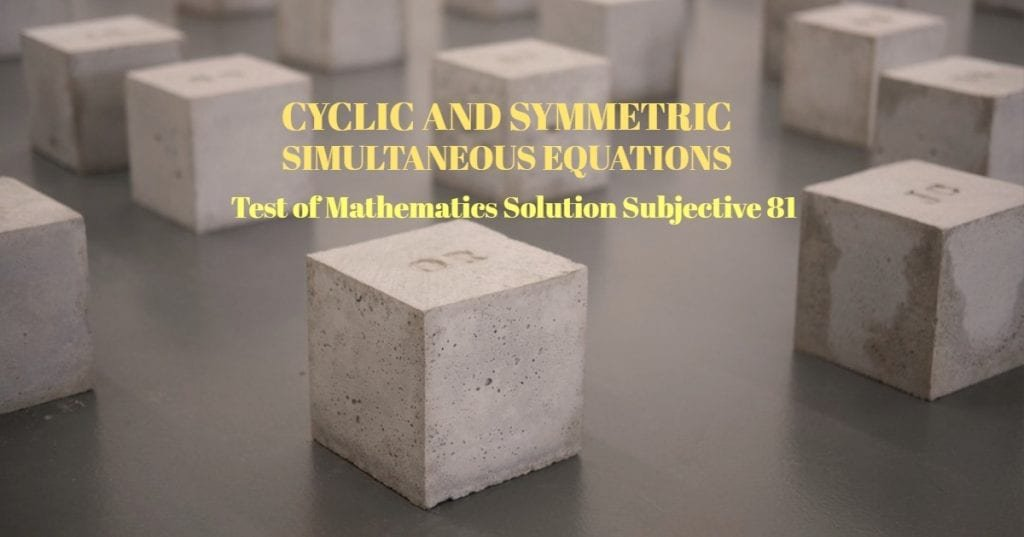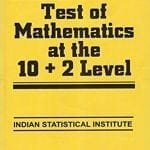This is a Test of Mathematics Solution Subjective 81 (from ISI Entrance). The book, Test of Mathematics at 10+2 Level is Published by East West Press. This problem book is indispensable for the preparation of I.S.I. B.Stat and B.Math Entrance.

Also visit: I.S.I. & C.M.I. Entrance Course of Cheenta

## Problem

Find all possible real numbers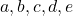which satisfy the following set of equations: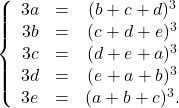## Solution

Observing the symmetry and the cyclicity of the given set of equations it can be easily inferred that the real numbers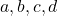and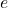cannot be ordered i.e. one number cannot be greater or smaller than the other number else the system of equations will be inconsistent. This can be shown easily with the help of inequality.

Without loss of generality, we can say  that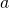is greater or equal to them all, i.e.,. Thus we have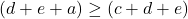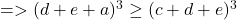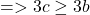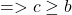As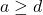, we also have,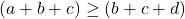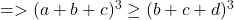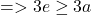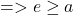Thus we have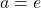Further calculations will show that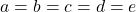is the only possible solution to this set of equations.

Thus we are left to solve just one equation, i.e.,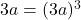which gives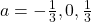Thus the possible values of the 5-tupleare: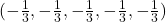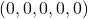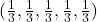This is a Test of Mathematics Solution Subjective 81 (from ISI Entrance). The book, Test of Mathematics at 10+2 Level is Published by East West Press. This problem book is indispensable for the preparation of I.S.I. B.Stat and B.Math Entrance.

Also visit: I.S.I. & C.M.I. Entrance Course of Cheenta

## Problem

Find all possible real numberswhich satisfy the following set of equations:## Solution

Observing the symmetry and the cyclicity of the given set of equations it can be easily inferred that the real numbersandcannot be ordered i.e. one number cannot be greater or smaller than the other number else the system of equations will be inconsistent. This can be shown easily with the help of inequality.

Without loss of generality, we can say  thatis greater or equal to them all, i.e.,. Thus we haveAs, we also have,Thus we haveFurther calculations will show thatis the only possible solution to this set of equations.

Thus we are left to solve just one equation, i.e.,which givesThus the possible values of the 5-tupleare:### Knowledge Partner